# ggplot2: Legend - Part 4

### Introduction

This is the 16th post in the series Elegant Data Visualization with ggplot2. In the previous post, we learnt how to modify the legend of plot when `shape` is mapped to categorical variables. In this post, we will learn to modify the following using `scale_size_continuous` when `size` aesthetic is mapped to variables:

• title
• breaks
• limits
• range
• labels
• values

### Libraries, Code & Data

We will use the following libraries in this post:

All the data sets used in this post can be found here and code can be downloaded from here.

### Plot

Let us start with a scatter plot examining the relationship between displacement and miles per gallon from the mtcars data set. We will map the size of the points to the `hp` variable. Remember, `size` must always be mapped to a continuous variable.

``````ggplot(mtcars) +
geom_point(aes(disp, mpg, size = hp))``````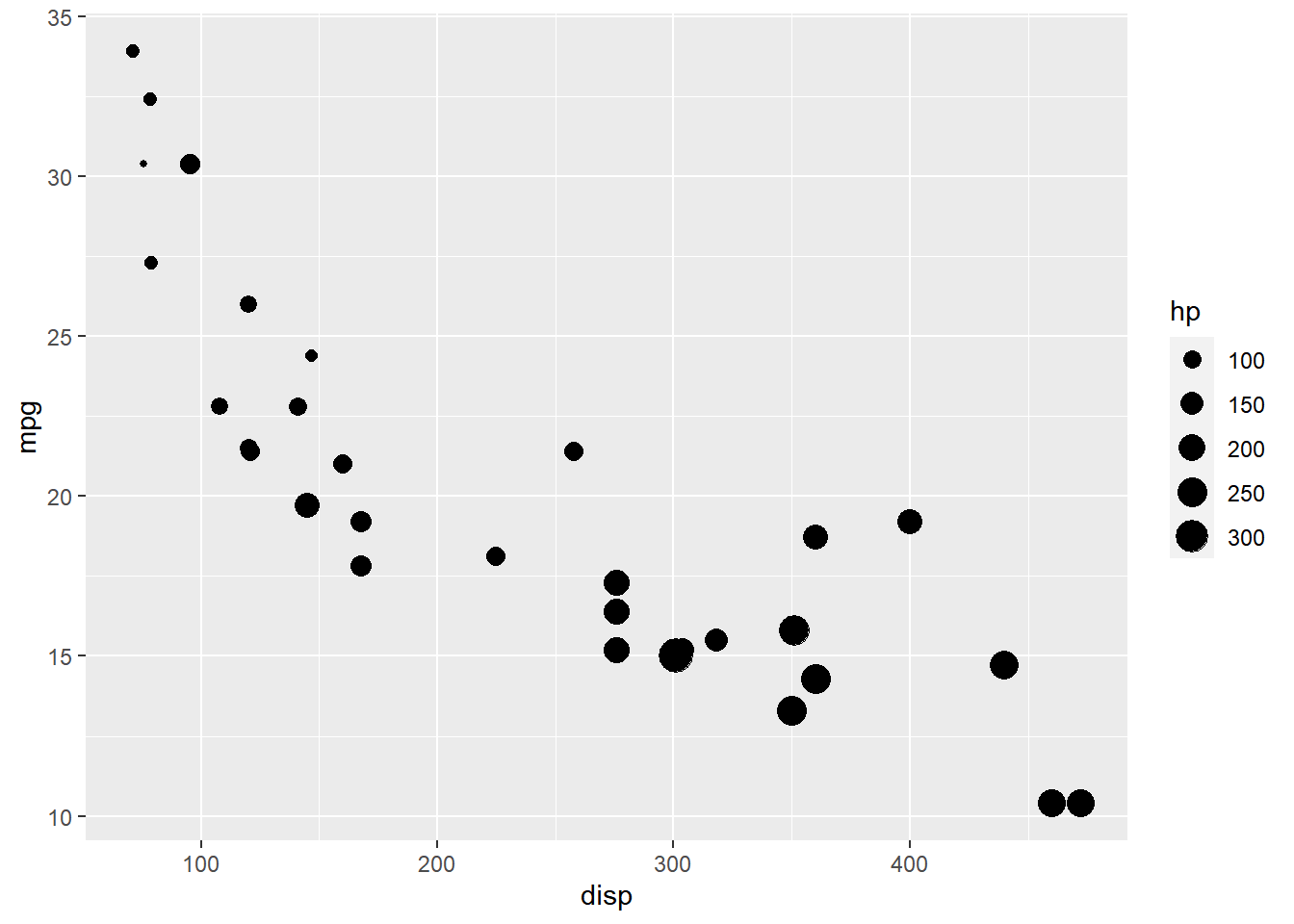As you can see, the legend acts as a guide for the `size` aesthetic. Now, let us learn to modify the different aspects of the legend.

### Title

The title of the legend (`hp`) is not very intuitive. If the user does not know the underlying data, they will not be able to make any sense out of it. Let us change it to `Horsepower` using the `name` argument.

``````ggplot(mtcars) +
geom_point(aes(disp, mpg, size = hp)) +
scale_size_continuous(name = "Horsepower")``````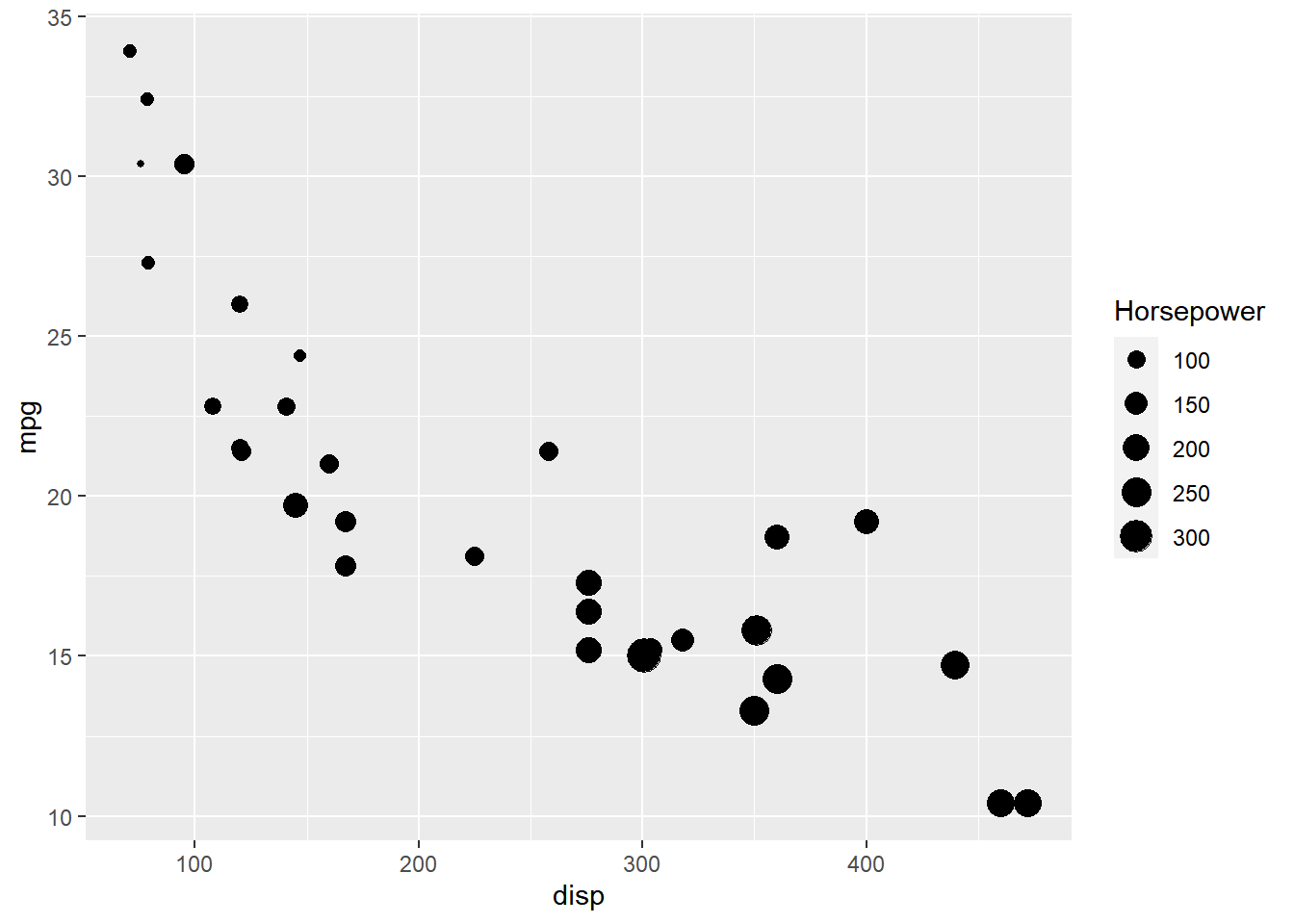### Range

The range of the size of points can be modified using the `range` argument. We need to specify a lower and upper range using a numeric vector. In the below example, we use `range` and supply the lower and upper limits as `3` and `6`. The size of the points will now lie between `3` and `6` only.

``````ggplot(mtcars) +
geom_point(aes(disp, mpg, size = hp)) +
scale_size_continuous(range = c(3, 6))``````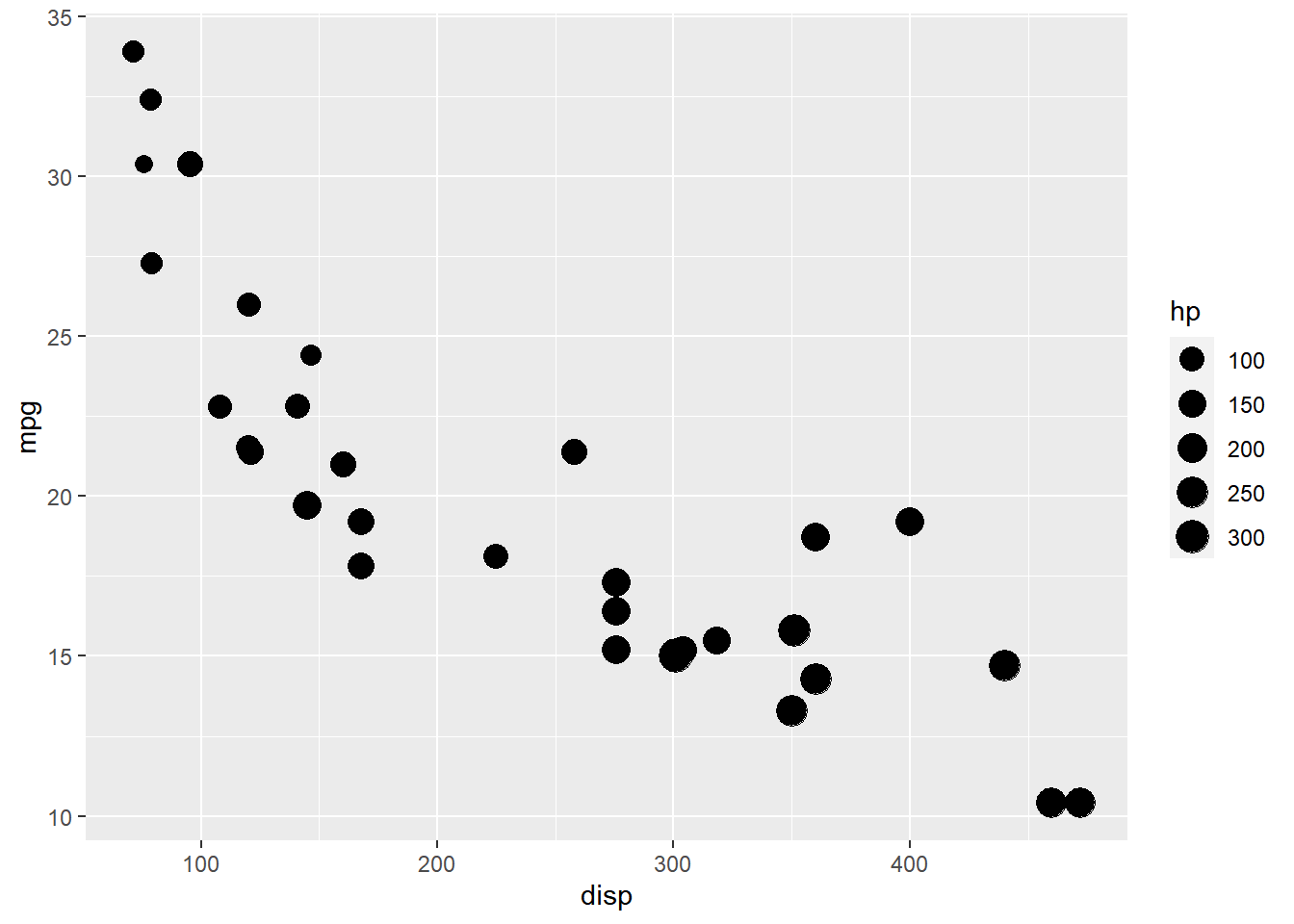### Limits

Let us assume that we want to modify the data to be displayed i.e. instead of examining the relationship between mileage and displacement for all cars, we desire to look at only cars whose horsepower is between `100` and `350`. One way to approach this would be to filter the data using `filter` from dplyr and then visualize it. Instead, we will use the `limits` argument and filter the data for visualization.

``````ggplot(mtcars) +
geom_point(aes(disp, mpg, size = hp)) +
scale_size_continuous(limits = c(100, 350))``````
``## Warning: Removed 9 rows containing missing values (geom_point).``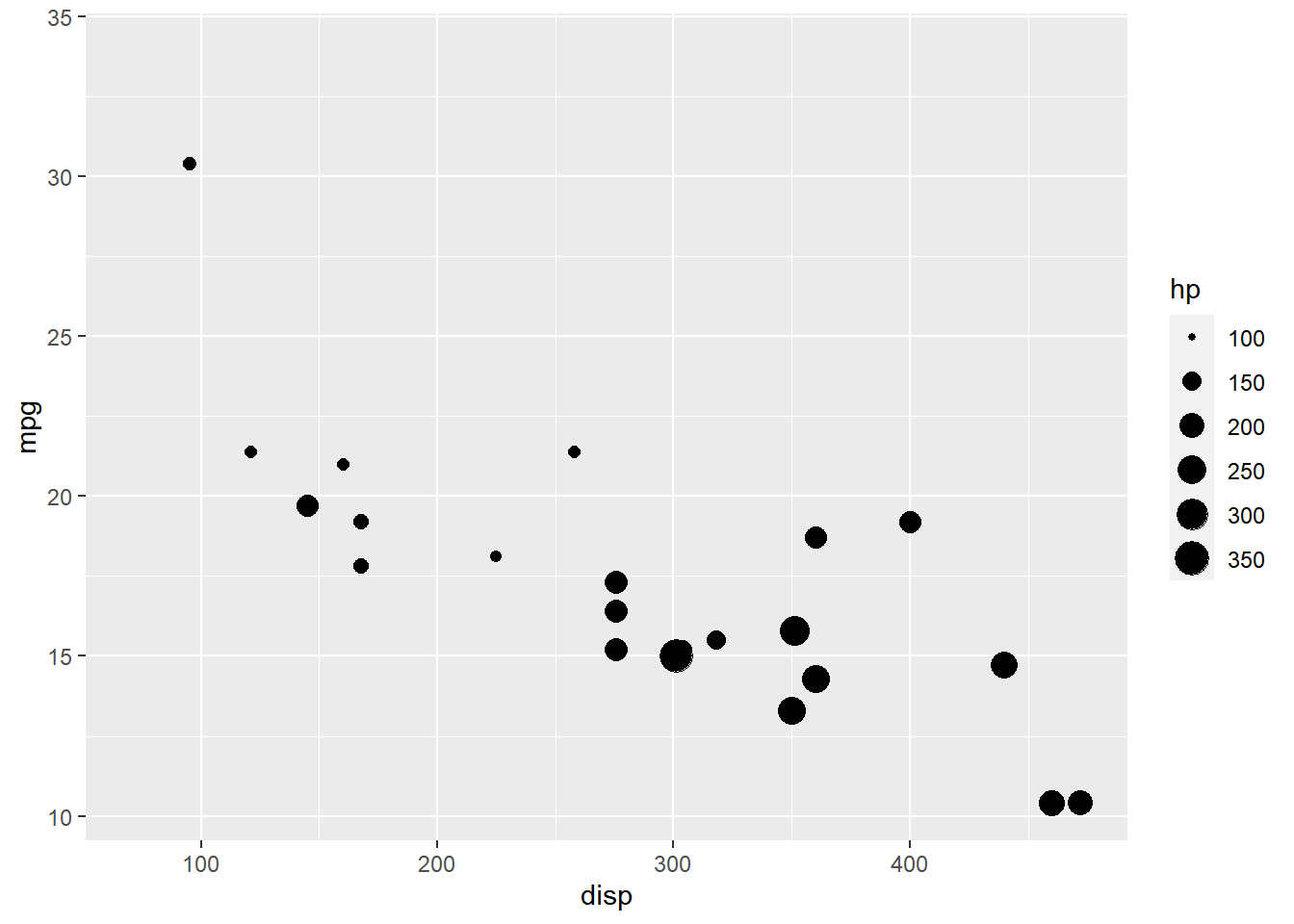### Breaks

When the range of the variable mapped to size is large, you may not want the labels in the legend to represent all of them. In such cases, we can use the breaks argument and specify the labels to be used. In the below case, we use the `breaks` argument to ensure that the labels in legend represent certain midpoints (125, 200, 275) of the mapped variable.

``````ggplot(mtcars) +
geom_point(aes(disp, mpg, size = hp)) +
scale_size_continuous(breaks = c(125, 200, 275))``````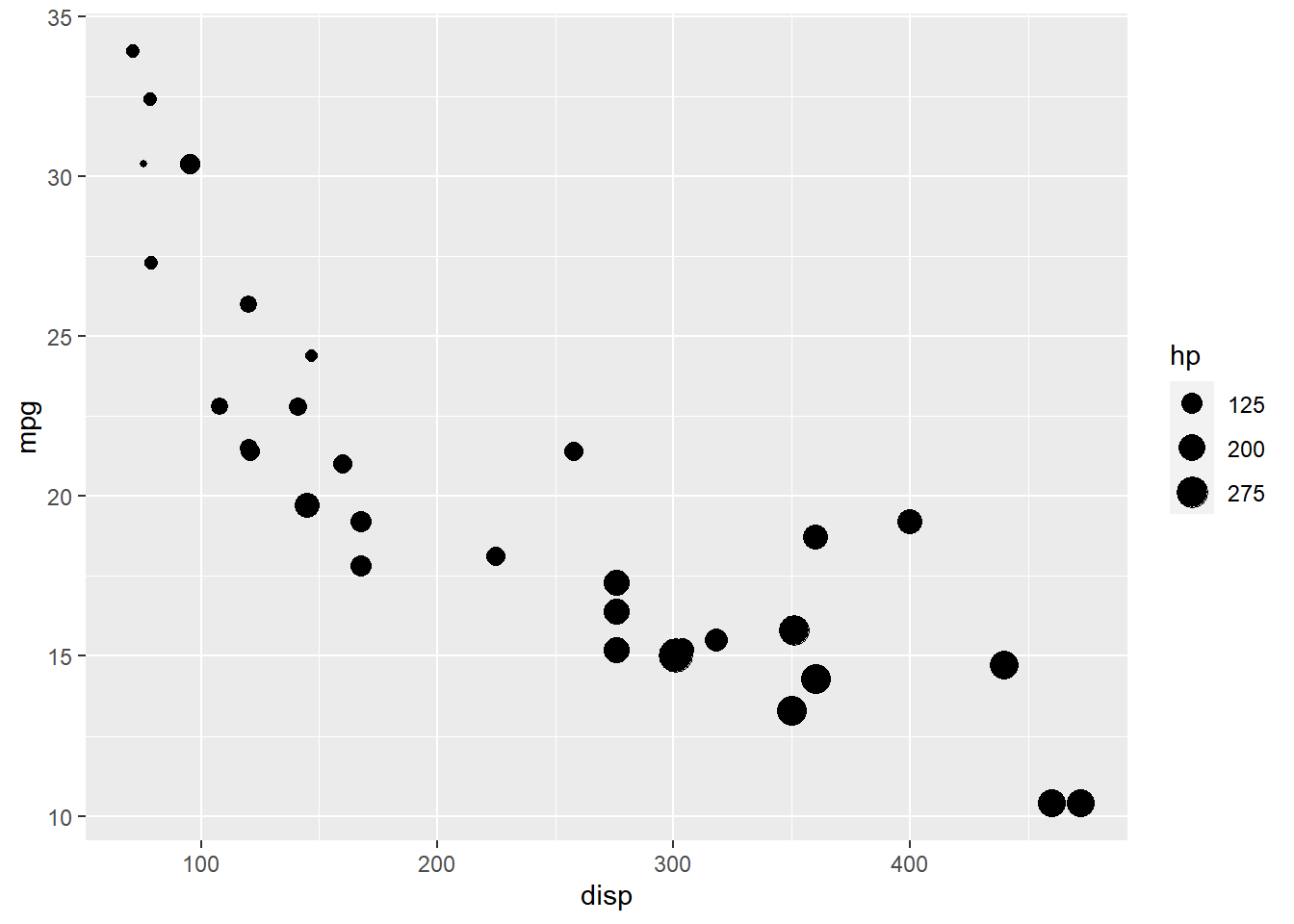### Labels

The labels in the legend can be modified using the `labels` argument. Let us change the labels to “1 Hundred”, “2 Hundred” and “3 Hundred” in the next example. Ensure that the labels are intuitive and easy to interpret for the end user of the plot.

``````ggplot(mtcars) +
geom_point(aes(disp, mpg, size = hp)) +
scale_size_continuous(breaks = c(100, 200, 300),
labels = c("1 Hundred", "2 Hundred", "3 Hundred"))``````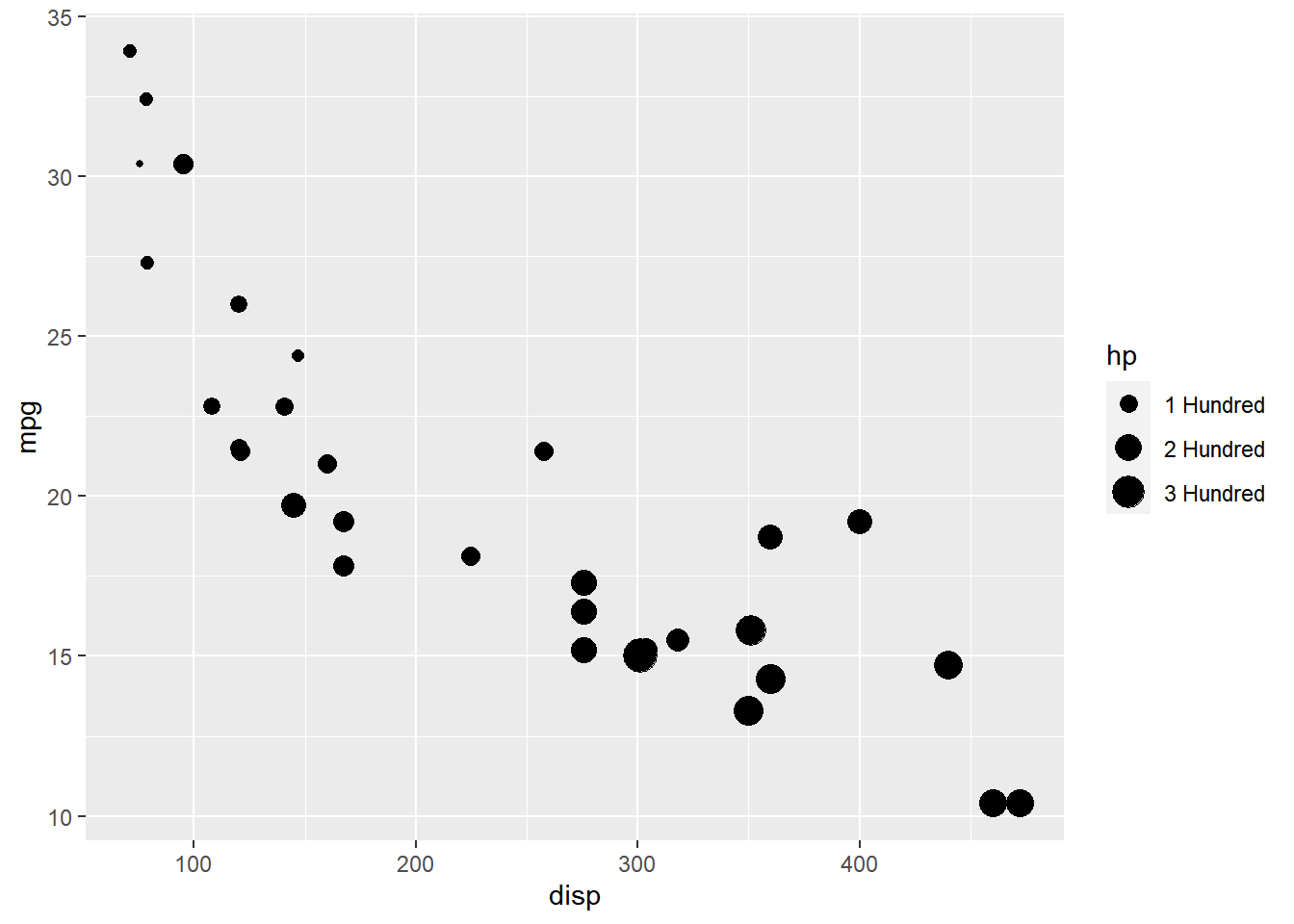### Putting it all together

``````ggplot(mtcars) +
geom_point(aes(disp, mpg, size = hp)) +
scale_size_continuous(name = "Horsepower", range = c(3, 6),
limits = c(0, 400), breaks = c(100, 200, 300),
labels = c("1 Hundred", "2 Hundred", "3 Hundred"))``````### Summary

In this post, we learnt to modify the following aspects of legends:

• title
• breaks
• range
• limits
• labels
• values

### Up Next..

In the next post, we will learn to modify the legend when `alpha` is mapped to variables.